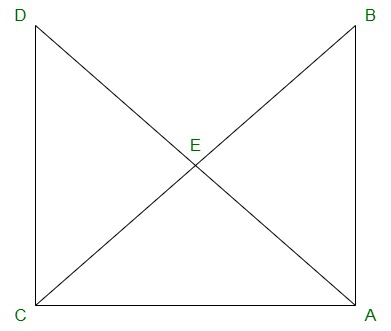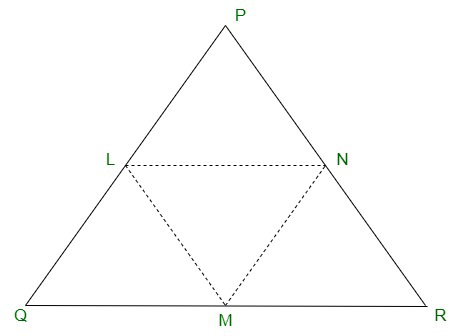Related Articles

# Class 9 RD Sharma Solutions – Chapter 10 Congruent Triangles- Exercise 10.4

• Last Updated : 11 Dec, 2020

### Question 1: In figure, It is given that AB = CD and AD = BC. Prove that ΔADC ≅ ΔCBA.Solution:

Given:
AB = CD and AD = BC.

To prove:

AB = CD {Given}
And AC = AC {Common side}
So,
By SSS congruence criterion, we have

Hence, proved.

### Question 2: In a ΔPQR, if PQ = QR and L, M and N are the mid-points of the sides PQ, QR and RP respectively. Prove that LN = MN.

Solution:Given:

In Δ PQR, PQ = QR and L, M and N are the mid-points of the sides PQ, QR and RP respectively

To prove:
LN = MN

Join L and M, M and N, N and L
We have PL = LQ, QM = MR and RN = NP
[Since, L, M and N are mid-points of PQ, QR and RP respectively]
And also PQ = QR
PL = LQ = QM = MR = PN = LR —->(equation 1)

MN || PQ and MN =  PQ    { Using mid-point theorem}
2
MN = PL = LQ —->(equation 2)

Similarly, we have
LN || QR and LN =  QR
2
LN = QM = MR —->(equation 3)

From (equation 1), (equation 2) and (equation 3),
We have
PL = LQ = QM = MR = MN = LN
This implies, LN = MN

Hence, Proved.

Attention reader! All those who say programming isn’t for kids, just haven’t met the right mentors yet. Join the  Demo Class for First Step to Coding Coursespecifically designed for students of class 8 to 12.

The students will get to learn more about the world of programming in these free classes which will definitely help them in making a wise career choice in the future.

My Personal Notes arrow_drop_up